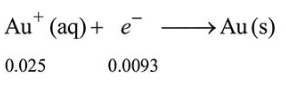# 250mL of a waste solution obtained from the workshop ofQuestion:

$250 \mathrm{~mL}$ of a waste solution obtained from the workshop of a goldsmith contains $0.1 \mathrm{M} \mathrm{AgNO}_{3}$ and $0.1 \mathrm{M} \mathrm{AuCl}$. The solution was electrolyzed at $2 \mathrm{~V}$ by passing a current of 1 A for 15 minutes. The metal/metals electrodeposited will be :

$\left(\mathrm{E}_{\mathrm{Ag}^{+} / \mathrm{Ag}}^{0}=0.80 \mathrm{~V}, \mathrm{E}_{\mathrm{Au}^{+} / \mathrm{Au}}^{0}=1.69 \mathrm{~V}\right)$

1. only gold

2. silver and gold in proportion to their atomic weights

3. only silver

4. silver and gold in equal mass proportion

Correct Option: 1

Solution:

Millimoles of $\mathrm{Au}^{+}=0.1 \times 250=25$

Mole of $\mathrm{Au}^{+}=\frac{25}{1000}=\frac{1}{40}=0.025$

Similarly, moles of $\mathrm{Ag}^{+}=0.025$

Charge passed $=\mathrm{I} \times t=1 \times 15 \times 60=900 \mathrm{C}$

Moles of $\mathrm{e}^{-}$passed $=\frac{900}{96500}=0.0093 \mathrm{~mol}$.

Species with higher value of SRP will get deposited first at cathode.So, only Au will get deposited.